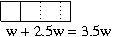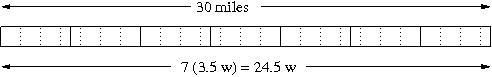# Morning Walk

Alignments to Content Standards: 6.EE.B.7

Sierra walks her dog Pepper twice a day. Her evening walk is two and a half times as far as her morning walk. At the end of the week she tells her mom,

I walked Pepper for 30 miles this week!

How long is her morning walk?

## IM Commentary

This task presents a straight forward question that can be solved using an equation in one variable. The numbers are complicated enough so that it is natural to set up an equation rather than solve the problem in one's head.

An instructor could use 6.EE Busy Day to introduce the idea of using variables to represent unknown quantities and follow up with 6.EE Morning Walk where the algebraic approach is both grade-appropriate and clearly more efficient to help students see the value of a symbolic approach.

## Solutions

Solution: Solving an equation

If we let $w$ denote the length of the morning walk, Sierra walks $w+2.5w$ or $3.5w$ miles each day. At the end of the week she has walked 7 times as far and she said that this was 30 miles. Solving the equation $$24.5w = 30,$$ we have $w=30/24.5 \approx 1.2$ miles. Therefore the distance of Sierra's morning walk is about 1.2 miles

Solution: Using a diagram

We can illustrate the solution to this problem with a tape diagram. If we let one box be the morning walk, then the evening walk is $2.5$ boxes. The total distance Sierra walks in one day is represented by $3.5$ boxes. Instead of talking about the number of boxes, we can choose a variable to represent the value or length of each box, say $w$.Since Sierra walks every day of the week, the total distance walked is $7$ times as long. Therefore, we have the total distance walked during the week is $7(3.5w) = 24.5 w.$ We know that Sierra walks a total distance of 30 miles, so we have $24.5 w = 30$ miles. We can use another diagram to illustrate the total distance with $24.5$ boxes.Dividing both sides of the equation $24.5w=30$ by 24.5 allows us to find $w,$ and we have

$$w = 30/24.5 \approx 1.2.$$

Therefore, Sierra's morning walk is about 1.2 miles.